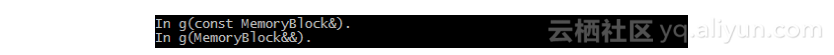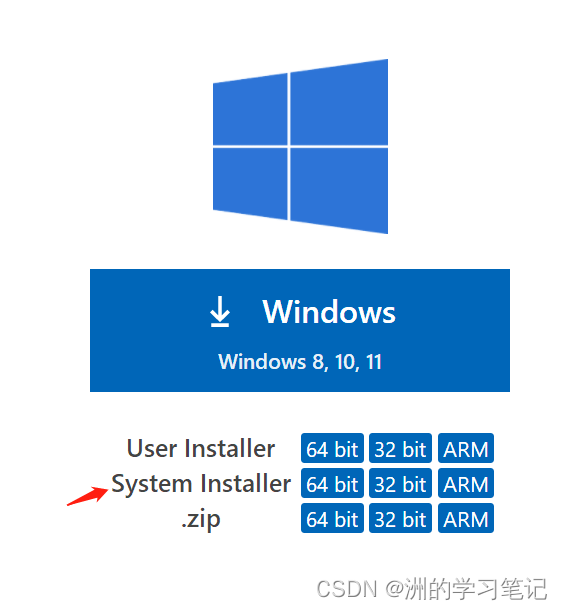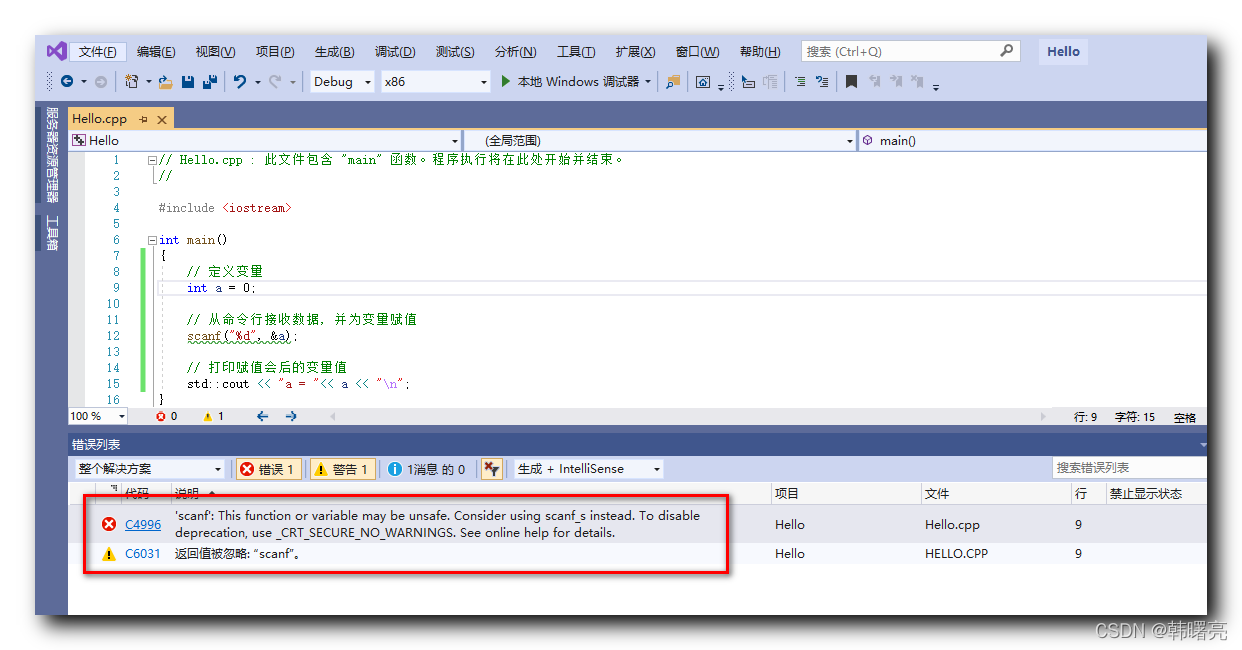# 《Visual C++ 2012 开发权威指南》——2.2　Visual C++2012的语言新特性（2）

+关注继续查看

### 2.2　Visual C++2012的语言新特性（2）

Rvalue引用：N1610"Rvalues类对象的初始化的澄清"是早期尝试启用无rvalue引用move　 语意。

Rvalue引用v3.0添加自动生成的构造函数和移动赋值运算符在一定条件下的新规则。这不会进行中VC11，还将继续遵循的永远不会自动生成move构造函数/移动页本页的行为。

Rvalue引用支持移动语义的实现，可以显著提高应用程序的性能。移动语义使能够调用资源编写代码（如动态分配的内存）来自对象到另一个。移动语义工作，因为它可以使资源从一个程序不能在其他位置引用的临时对象。

// string_concatenation.cpp
// compile with: /EHsc
#include <iostream>
#include <string>
using namespace std;

int main()
{
string s = string("h") +"e" +"ll" +"o";
cout << s << endl;
}

struct W
{
W(int&, int&) {}
};

struct X
{
X(const int&, int&) {}
};

struct Y
{
Y(int&, const int&) {}
};

struct Z
{
Z(const int&, const int&) {}
};

template <typename T, typename A1, typename A2>
T* factory(A1& a1, A2& a2)
{
return new T(a1, a2);
}

int a = 4, b = 5;
W* pw = factory(a, b);

Z* pz = factory<Z>(2, 2);

template <typename T, typename A1, typename A2>
T* factory(A1&& a1, A2&& a2)
{
return new T(std::forward<A1>(a1), std::forward<A2>(a2));
}

int main()
{
int a = 4, b = 5;
W* pw = factory(a, b);
X* px = factory(2, b);
Y* py = factory(a, 2);
Z* pz = factory(2, 2);

delete pw;
delete px;
delete py;
delete pz;
}
Rvalue其他属性引用

// reference-overload.cpp
// Compile with: /EHsc
#include <iostream>
using namespace std;

// 使用内存资源的类
class MemoryBlock
{
// 在此处为该类添加资源
};

void f(const MemoryBlock&)
{
cout << "In f(const MemoryBlock&)．This version cannot modify the parameter." << endl;
}

void f(MemoryBlock&&)/
{
cout << "In f(MemoryBlock&&)．This version can modify the parameter." << endl;
}

int main()
{
MemoryBlock block;
f(block);
f(MemoryBlock());
}

![image](https://yqfile.alicdn.com/7dd83c3ff7e409e1b5a9af522c8d2d82f2b2c0a2.png)

// named-reference.cpp
// Compile with: /EHsc

# include

using namespace std;

//使用内存资源的类.
class MemoryBlock
{
//在此处为该类添加资源.
};

void g(const MemoryBlock&)
{
cout << "In g(const MemoryBlock&)." << endl;
}

void g(MemoryBlock&&)
{
cout << "In g(MemoryBlock&&)." << endl;
}

MemoryBlock&& f(MemoryBlock&& block)
{
g(block);
return block;
}

int main()
{
g(f(MemoryBlock()));
}STL std:: 移动功能可以转换为rvalue的对象对该对象的引用。或者，如下面的示例所示，可以使用static_cast关键字左值转换为rvalue引用，例如：

// cast-reference.cpp
// Compile with: /EHsc
#include <iostream>
using namespace std;

//使用内存资源的类
class MemoryBlock
{
//在此处为该类添加资源.
};

void g(const MemoryBlock&)
{
cout << "In g(const MemoryBlock&)." << endl;
}

void g(MemoryBlock&&)
{
cout << "In g(MemoryBlock&&)." << endl;
}

int main()
{
MemoryBlock block;
g(block);
g(static_cast<MemoryBlock&&>(block));
}

![image](https://yqfile.alicdn.com/ba513c17d25b4eb0fb6e480861450fdce524eb27.png)

// template-type-deduction.cpp
// Compile with: /EHsc

# include

using namespace std;

template struct S;

// 下面分别制定S为
// lvalue reference (T&), const lvalue reference (const T&),
// rvalue reference (T&&), const rvalue reference (const T&&).
// 每个结构提供输出函数来输出每个结构的参数和类型.

template struct S {
static void print(T& t)
{
cout << "print: " << t << endl;
}
};

template struct S {
static void print(const T& t)
{
cout << "print: " << t << endl;
}
};

template struct S {
static void print(T&& t)
{
cout << "print: " << t << endl;
}
};

template struct S {
static void print(const T&& t)
{
cout << "print: " << t << endl;
}
};

template void print_type_and_value(T&& t)
{
S::print(std::forward(t));
}

// 此函数返回字符串 "fourth".
const string fourth() { return string("fourth"); }

int main()
{

string s1("first");
print_type_and_value(s1);

const string s2("second");
print_type_and_value(s2);

// 下面的调用可以解析为：print_type_and_value(string&& t)
print_type_and_value(string("third"));

//下面的调用可以解析为：print_type_and_value(const string&& t)
print_type_and_value(fourth());
}print_type_and_value<string&>(string& && t)

print_type_and_value<string&>(string& t)
print_type_and_value功能的此版本向前然后它对S::print方法的正确的专用版本的参数。C++ Visual Studio 2022 中的改进、行为更改和错误修复
C++ Visual Studio 2022 中的改进、行为更改和错误修复
20 0Visual Studio 2022 版本 17.4 预览版 3 中对c++编译时优化的内容你都知道吗
Visual Studio 2022 版本 17.4 预览版 3 中对c++编译时优化的内容你都知道吗
9 0Visual Studio如何调用已配置过的C++库？

76 0122 0Linux Ubuntu配置Visual Studio Code及C++环境的方法

286 00基础都能看懂的 Visual Studio Code(VScode)使用脚本一键配置安装C/C++环境、编译运行Windows版本教程（内附脚本、安装包下载链接）

133 0157 0【错误记录】Visual Studio 编译 C++ 代码报错 ( To disable deprecation, use _CRT_SECURE_NO_WARNINGS. )
【错误记录】Visual Studio 编译 C++ 代码报错 ( To disable deprecation, use _CRT_SECURE_NO_WARNINGS. )
424 0

C++ 入门教程开发文档1729442

GPON Class C++ SFP OLT Transce